### RSLTexture Channels

#### Introduction

This note shows how 5 texture maps can be "packed" into a five channel image - one map per channel. Figure 1 shows a five channel PhotoShop image. The last two channels have black backgrounds because PhotoShop considers them to be alpha or transparency channels.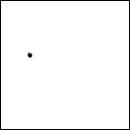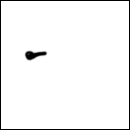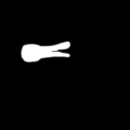Figure 1

When applied by the shader shown in listing 1 the maps can provide the illusion of, say the discoloration of the surface of a fruit, becoming larger over time. The shader simply blends from one map channel into another - figures 1 and 2.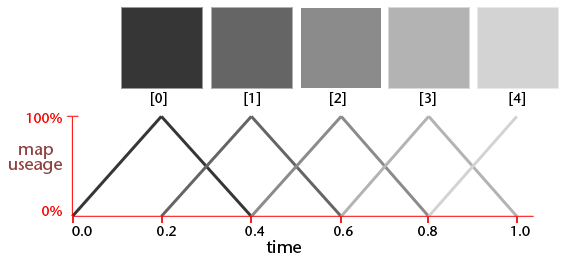Figure 2
Progressive use of a 5 channel map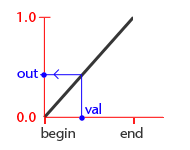Figure 3
The straightstep function uses simple linear interpolation

The five channel PhotoShop image was saved as a tif file and converted to a texture using Pixar's txmake utility.

Listing 1

 ```float straightstep(float begin, end, val) { float out; if(val <= begin) out = 0; else if(val >= end) out = 1; else out = (val - begin)/(end - begin); return 1; } //------------------------------------------ surface blotch(float Kd = 1, ime = 0; string map = ""; color blotch_color = 0) { color surfcolor = 1; normal n = normalize(N); normal nf = faceforward(n, I); float tex; if(map != "") { if(time <= 0.20) tex = mix(1, texture(map), smoothstep(0,0.20,time)); else if(time > 0.20 && time <= 0.4) tex = mix(texture(map), texture(map), smoothstep(0.2,0.4,time)); else if(time > 0.4 && time <= 0.6) tex = mix(texture(map), texture(map), smoothstep(0.4,0.6,time)); else if(time > 0.6 && time <= 0.8) tex = mix(texture(map), 1 - texture(map), smoothstep(0.6,0.8,time)); else if(time > 0.8) tex = mix(texture(map), 1 - texture(map), smoothstep(0.8,1.0,time)); // composite the texture map color over the background surfcolor = mix(blotch_color, Cs, tex); } Oi = Os; Ci = Oi * Cs * surfcolor * Kd * diffuse(nf); }```

Slightly different results can be obtained by substituting the RSL function `smoothstep`() with the custom `straightstep`() function.